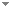Switch to: References

# Prefix and plain Kolmogorov complexity characterizations of 2-randomness: simple proofs

Archive for Mathematical Logic 54 (5-6):615-629 (2015)

 Randomness Notions and Reverse Mathematics.André Nies & Paul Shafer - 2020 - Journal of Symbolic Logic 85 (1):271-299.We investigate the strength of a randomness notion ${\cal R}$ as a set-existence principle in second-order arithmetic: for each Z there is an X that is ${\cal R}$-random relative to Z. We show that the equivalence between 2-randomness and being infinitely often C-incompressible is provable in $RC{A_0}$. We verify that $RC{A_0}$ proves the basic implications among randomness notions: 2-random $\Rightarrow$ weakly 2-random $\Rightarrow$ Martin-Löf random $\Rightarrow$ computably random $\Rightarrow$ Schnorr random. Also, over $RC{A_0}$ the existence of computable randoms is equivalent (...) Direct download (2 more)     Export citationBookmark